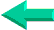This is an excerpt from Pierre Leclerc (www.excel-vba.com)

The 2 Most Important

Excel Functions AND
Formulas

SUMPRODUCT

AND

INDEX/MATCH

## Annex 4: Excel Spreadsheets Financial Functions (53)

There are 53 functions in the "Financial" category.

 Functions What it Does FV Returns the future value of an investment NPER Returns the number of periods for an investment PMT Returns the periodic payment for an annuity RATE Returns the interest rate per period of an annuity ACCRINT Returns the accrued interest for a security that pays periodic interest ACCRINTM Returns the accrued interest for a security that pays interest at maturity AMORDEGRC Returns the depreciation for each accounting period by using a depreciation coefficient AMORLINC Returns the depreciation for each accounting period COUPDAYBS Returns the number of days from the beginning of the coupon period to the settlement date COUPDAYS Returns the number of days in the coupon period that contains the settlement date COUPDAYSNC Returns the number of days from the settlement date to the next coupon date COUPNCD Returns the next coupon date after the settlement date COUPNUM Returns the number of coupons payable between the settlement date and maturity date COUPPCD Returns the previous coupon date before the settlement date CUMIPMT Returns the cumulative interest paid between two periods CUMPRINC Returns the cumulative principal paid on a loan between two periods DB Returns the depreciation of an asset for a specified period using the fixed-declining balance method DDB Returns the depreciation of an asset for a specified period using the double-declining balance method or some other method you specify DISC Returns the discount rate for a security DOLLARDE Converts a dollar price, expressed as a fraction, into a dollar price, expressed as a decimal number DOLLARFR Converts a dollar price, expressed as a decimal number, into a dollar price, expressed as a fraction DURATION Returns the annual duration of a security with periodic interest payments EFFECT Returns the effective annual interest rate FVSCHEDULE Returns the future value of an initial principal after applying a series of compound interest rates INTRATE Returns the interest rate for a fully invested security IPMT Returns the interest payment for an investment for a given period IRR Returns the internal rate of return for a series of cash flows ISPMT Returns the interest paid during a specific period of an investment MDURATION Returns the Macauley modified duration for a security with an assumed par value of \$100 MIRR Returns the internal rate of return where positive and negative cash flows are financed at different rates NOMINAL Returns the annual nominal interest rate NPV Returns the net present value of an investment based on a series of periodic cash flows and a discount rate ODDFPRICE Returns the price per \$100 face value of a security with an odd first period ODDFYIELD Returns the yield of a security with an odd first period ODDLPRICE Returns the price per \$100 face value of a security with an odd last period ODDLYIELD Returns the yield of a security with an odd last period PPMT Returns the payment on the principal for an investment for a given period PRICE Returns the price per \$100 face value of a security that pays periodic interest PRICEDISC Returns the price per \$100 face value of a discounted security PRICEMAT Returns the price per \$100 face value of a security that pays interest at maturity PV Returns the present value of an investment RECEIVED Returns the amount received at maturity for a fully invested security SLN Returns the straight-line depreciation of an asset for one period SYD Returns the sum-of-years' digits depreciation of an asset for a specified period TBILLEQ Returns the bond-equivalent yield for a Treasury bill TBILLPRICE Returns the price per \$100 face value for a Treasury bill TBILLYIELD Returns the yield for a Treasury bill VDB Returns the depreciation of an asset for a specified or partial period using a declining balance method XIRR Returns the internal rate of return for a schedule of cash flows that is not necessarily periodic XNPV Returns the net present value for a schedule of cash flows that is not necessarily periodic YIELD Returns the yield on a security that pays periodic interest YIELDDISC Returns the annual yield for a discounted security for example, a Treasury bill YIELDMAT Returns the annual yield of a security that pays interest at maturity

If you are using a version of Excel earlier than 2007 you need to activate the "Excel Analysis Toolpack" to make some of the functions above available.

SUMPRODUCT  ¦  INDEX/MATCH  ¦  SUBTOTAL  ¦  ISERROR  ¦  HLOOKUP  ¦  LOOKUP  ¦  VLOOKUP
AVERAGEIF  ¦  AVERAGEIFS ¦  COUNTIF  ¦  COUNTIFS  ¦  SUMIF  ¦  SUMIFS  ¦  IFERRORBack home